There are thousands of candies involved in this quiz!

# Estimation in Arithmetical Calculations

This Math quiz is called 'Estimation in Arithmetical Calculations' and it has been written by teachers to help you if you are studying the subject at middle school. Playing educational quizzes is a fabulous way to learn if you are in the 6th, 7th or 8th grade - aged 11 to 14.

It costs only \$12.50 per month to play this quiz and over 3,500 others that help you with your school work. You can subscribe on the page at Join Us

When performing calculations, it’s always good to carry out a common-sense check and have a rough idea of the answer. This is true even if you are working with a calculator, as it is easy to mis-type an entry! Put your estimating and approximation skills to the test in this middle school Math quiz.

Approximating is all about making the calculation easier, by rounding each number to 1 significant figure. Very often, you will then be able to do it in your head. Things can get a little bit trickier when division is involved – ideally, you want both the numerator and denominator to have a common factor, so that one is a multiple of the other and it will cancel down.

Working out an approximate answer will give you an indication of the size of the answer (this is often called the ‘ball park figure’).

If your approximation tells you the answer is in the hundreds, but your proper calculation gives an answer smaller than 1, then you know you have gone wrong somewhere. Engineering disasters have resulted from errors in calculations, so it is important to get it right!

Look for everyday opportunities to practice your estimating skills – what’s the total of your shopping basket, how much change would you get from a £20 note, how many MP3 downloads can I get from a £5 voucher… the list is endless!

Question 1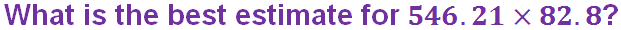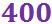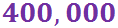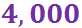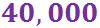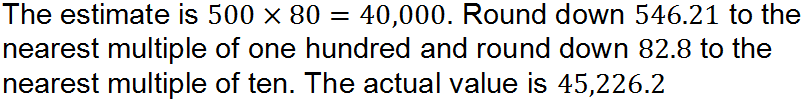Question 2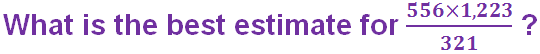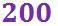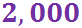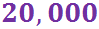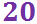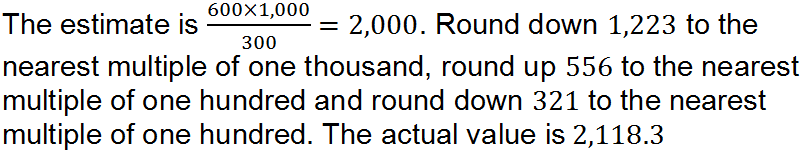Question 3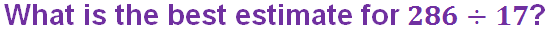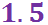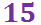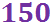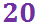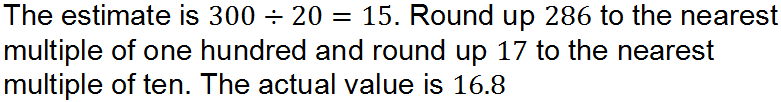Question 4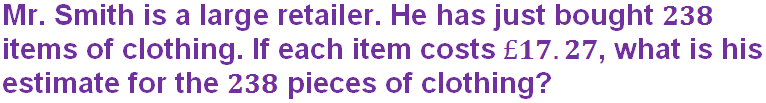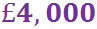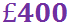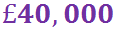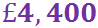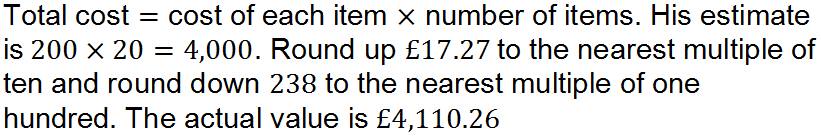Question 5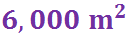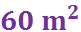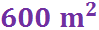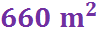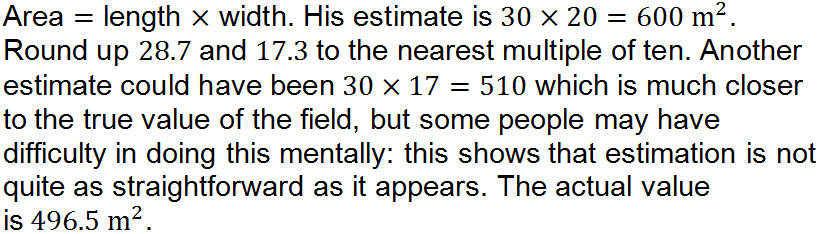Question 6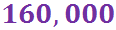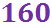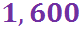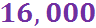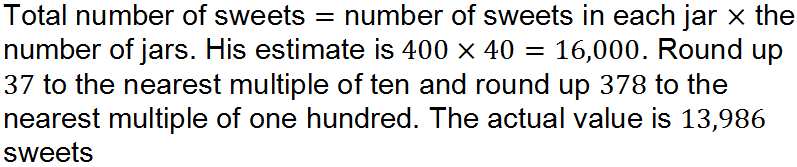Question 7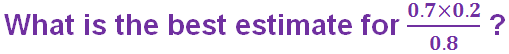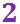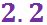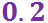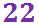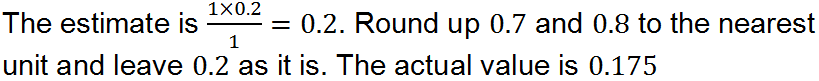Question 8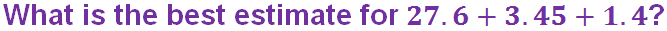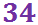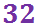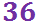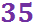Question 9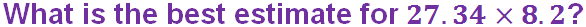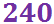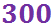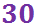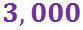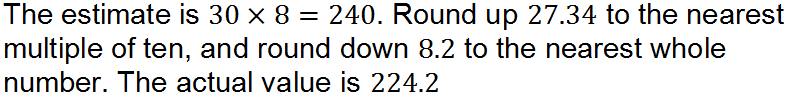Question 10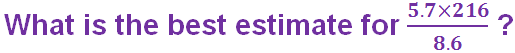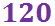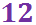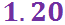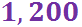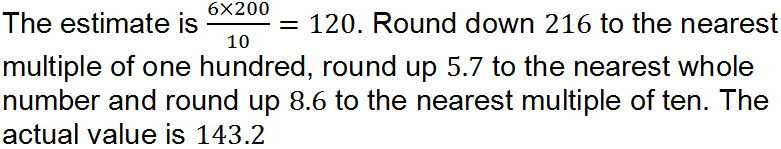Author:  Frank Evans